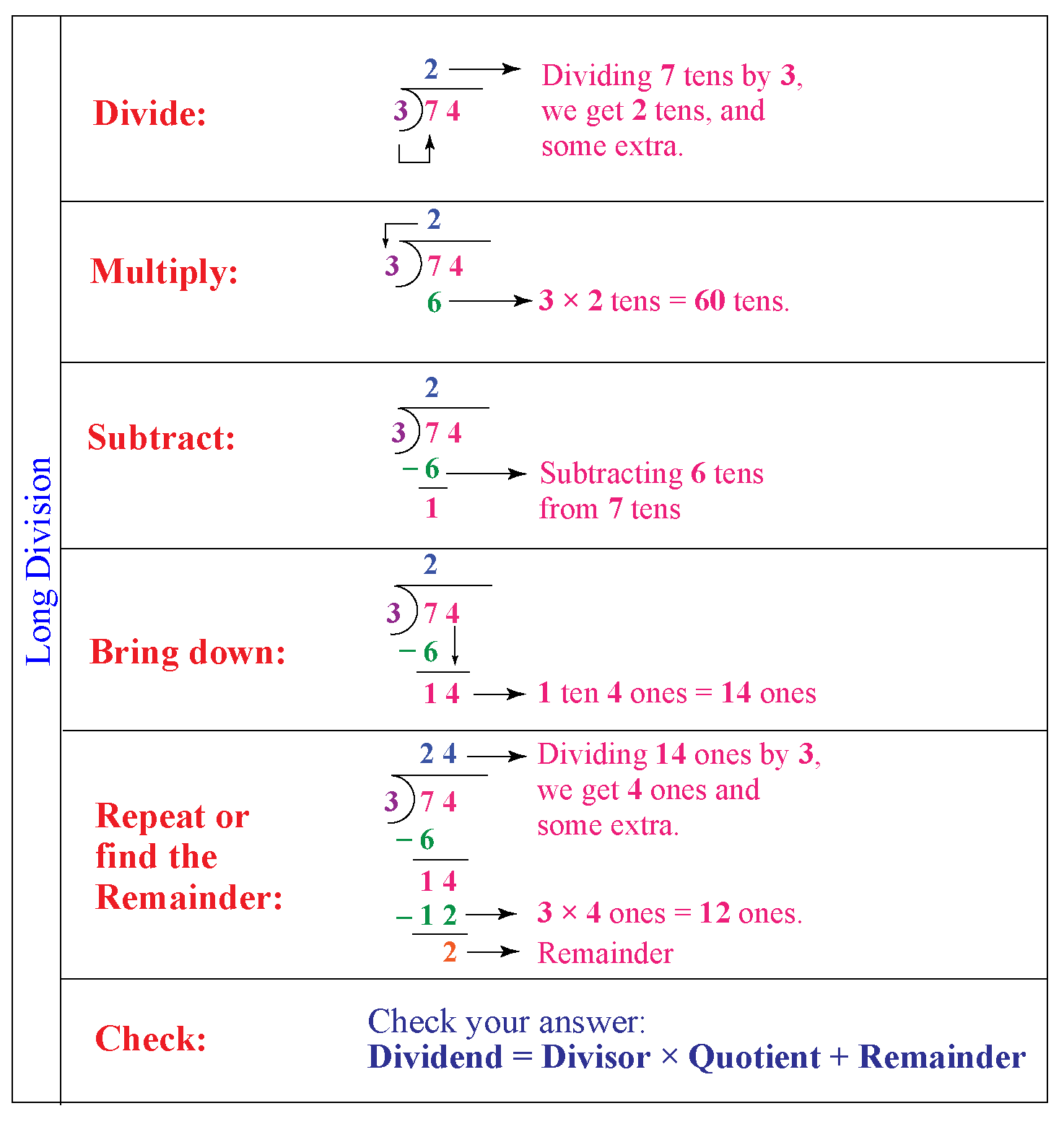# Long Division

Jane asked her teacher who gave the long division method we use today?

Her teacher said that the method we use today is an Italian method which was first described by Calandri in 1491

However, it was Henry Briggs, the first professor of Geometry, who named it as the 'long division method'.

Try this interactive calculator to get an idea about long division.

Enter the dividend and then the divisor.

Click on 'Calculate' to see the steps.

## Lesson Plan

 1 What Is Long Division Method? 2 Important Notes on Long Division 3 Tips and Tricks 4 Solved Examples on Long Division 5 Interactive Questions on Long Division

## What Is Long Division Method?

In Math, long division is a method for dividing large numbers into steps or parts, breaking the division problem into a sequence of easier steps.

## How to Do Long Division?

Division is one of the four basic mathematical operations, the other three being addition, subtraction and multiplication.

In arithmetic, long division is a standard division algorithm for dividing large numbers, breaking down a division problem into a series of easier steps.

It requires the constructs of a tableau.The divisor is separated from the dividend by a right parenthesis ⟨)⟩ or vertical bar ⟨|⟩ and the dividend is separated from the quotient by a vinculum (an overbar).

### Method

Long division steps for 74 $$\div$$ 3The same steps follow for long division problems related to long division polynomials and long division with decimals.

More Important Topics
Numbers
Algebra
Geometry
Measurement
Money
Data
Trigonometry
Calculus